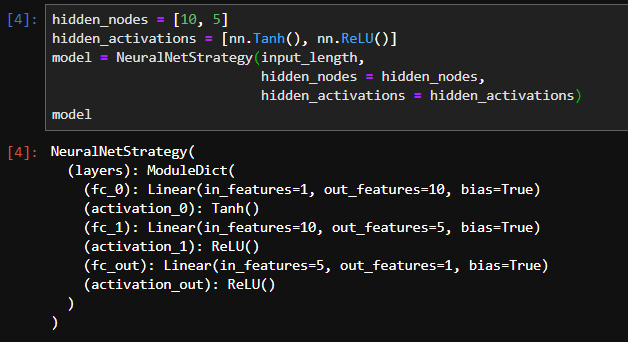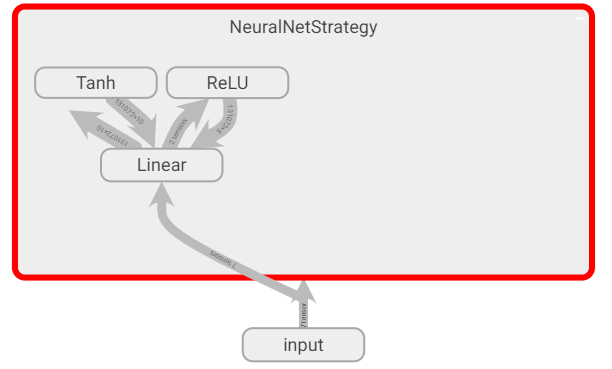# Named layers in tensorboard graph of dynamic Module

When I directly define a nn.Module with fixed layers and use `torch.utils.tensorboard.SummaryWriter`, I get a nice vizualisation of my computational graph like this::

``````
class NeuralNetStrategy(nn.Module):
def __init__(self, input_length):
super(NeuralNetStrategy, self).__init__()
self.fc1 = nn.Linear(input_length, 10)
self.fc_out = nn.Linear(10, 1)

def forward(self, x):
x = self.fc1(x).tanh()
x = self.fc_out(x).relu()
return x

model = NeuralNetStrategy(1)
with SummaryWriter(logdir) as writer:
``````

My goal is to get a similar output graph but for a dynamic number of layers created at runtime, but I’m having trouble to make this work.

I’ve tried using `nn.ModuleDict()` or building an OrderedDict and passing it to `nn.Sequential` in order to create named layers, but my tensorboard graph does not display the names. My current class definition and tensorboard output looks something like this:

``````class NeuralNetStrategy(nn.Module):
def __init__(self, input_length, hidden_nodes: Iterable[int], hidden_activations: Iterable):
super(NeuralNetStrategy, self).__init__()

self.layers = nn.ModuleDict()
self.layers['fc_0'] = nn.Linear(input_length, hidden_nodes)
self.layers['activation_0'] = hidden_activations

for i in range (1, len(hidden_nodes)):
self.layers['fc_' + str(i)] = nn.Linear(hidden_nodes[i-1], hidden_nodes[i])
self.layers['activation_' + str(i)] = hidden_activations[i]

self.layers['fc_out'] = nn.Linear(hidden_nodes[-1], 1)
self.layers['activation_out'] = nn.ReLU()

def forward(self, x):
for layer in self.layers.values():
x = layer(x)
return x
``````

When I initialize my model, it looks fine in pytorch (and does perform the desired computations), yet the tensorboard graph does not respect the named layers:How can I fix this?

1 Like